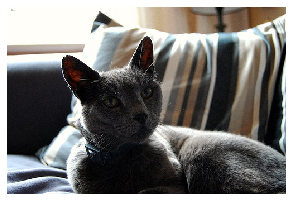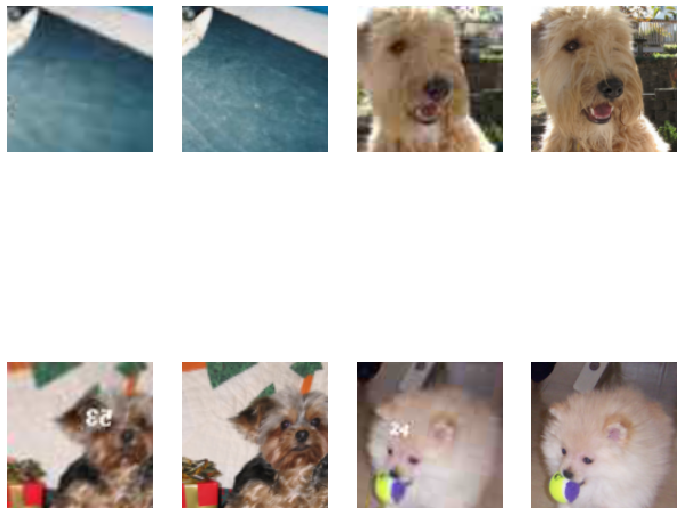## Lesson Video:

This article is also a Jupyter Notebook available to be run from the top down. There will be code snippets that you can then run in any environment.

Below are the versions of fastai, fastcore, and wwf currently running at the time of writing this:

• fastai: 2.1.10
• fastcore: 1.3.13
• wwf: 0.0.7

## What is a GAN?

The Generative Adversarial Network was invented by Ian Goodfellow, where two networks play a game. In our case, we will build a 'crappifier' to make images worse, and the critic will try to determine which is fake and which is the original. This will help us achieve super-resolution

from fastai.vision.all import *
from fastai.vision.gan import *


## Crappified data

Let's build a function first that will go through and 'crappify' some data:

from PIL import ImageDraw, ImageFont

def resize_to(img, targ_sz, use_min=False):
w,h = img.size
min_sz = (min if use_min else max)(w,h)
ratio = targ_sz/min_sz
return int(w*ratio),int(h*ratio)

class Crappifier():
"Quickly draw tesxt and numbers on an image"
def __init__(self, path_lr, path_hr):
self.path_lr = path_lr
self.path_hr = path_hr

def __call__(self, fn):
dest = self.path_lr/fn.relative_to(self.path_hr)
dest.parent.mkdir(parents=True, exist_ok=True)
img = Image.open(fn)
targ_sz = resize_to(img, 96, use_min=True)
img = img.resize(targ_sz, resample=Image.BILINEAR).convert('RGB')
w,h = img.size
q = random.randint(10,70)
ImageDraw.Draw(img).text((random.randint(0,w//2),random.randint(0,h//2)), str(q), fill=(255,255,255))
img.save(dest, quality=q)


And now let's get some data to work with. We'll use the PETS dataset:

path = untar_data(URLs.PETS)


We'll make two folders, one for the low resolution (LR) and high resolution (HR) photos:

path_hr = path/'images'
path_lr = path/'crappy'


Now let's generate our dataset!

items = get_image_files(path_hr)

parallel(Crappifier(path_lr, path_hr), items);


Let's take a look at one of our generated images:

bad_im = get_image_files(path_lr)

im1 = PILImage.create(items)

im1.show(); im2.show(figsize=(5,5))

<matplotlib.axes._subplots.AxesSubplot at 0x7fc44e2c1f28>Great! We have some data now! Let's build the DataBlock

## DataBlock

dblock = DataBlock(blocks=(ImageBlock, ImageBlock),
get_items=get_image_files,
get_y = lambda x: path_hr/x.name,
splitter=RandomSplitter(),
item_tfms=Resize(224),
batch_tfms=[*aug_transforms(max_zoom=2.),
Normalize.from_stats(*imagenet_stats)])


But for today's lesson, we'll go back to that progressive resizing technique we talked about. We want a function that can accept a batch_size and a im_size. Let's build that:

def get_dls(bs:int, size:int):
"Generates two GAN DataLoaders"
dblock = DataBlock(blocks=(ImageBlock, ImageBlock),
get_items=get_image_files,
get_y = lambda x: path_hr/x.name,
splitter=RandomSplitter(),
item_tfms=Resize(size),
batch_tfms=[*aug_transforms(max_zoom=2.),
Normalize.from_stats(*imagenet_stats)])
dls.c = 3 # For 3 channel image
return dls


## Pre-Trained Generator

The goal of this model will be to generate our "Super Resolution" images (or to make an image look better)

dls_gen = get_dls(32, 128)


On the left will be our 'crappified' image, and the right our original

dls_gen.show_batch(max_n=4, figsize=(12,12))Now let's build some models (with recommended hyper-parameters)

wd, y_range, loss_gen = 1e-3, (-3., 3.), MSELossFlat()


For our backbone, we'll use a resnet34

bbone = resnet34
cfg = unet_config(blur=True, norm_type=NormType.Weight, self_attention=True,
y_range=y_range)

def create_gen_learner():
return unet_learner(dls_gen, bbone, loss_func=loss_gen,
config=cfg)

learn_gen = create_gen_learner()

Downloading: "https://download.pytorch.org/models/resnet34-333f7ec4.pth" to /root/.cache/torch/checkpoints/resnet34-333f7ec4.pth


Let's fit our model quickly (we don't need to train for very long)

learn_gen.fit_one_cycle(2, pct_start=0.8, wd=wd)

epoch train_loss valid_loss time
0 0.072490 0.056544 01:03
1 0.039271 0.039441 00:57

Let's unfreeze and fit a bit more!

learn_gen.unfreeze()

learn_gen.fit_one_cycle(3, slice(1e-6,1e-3), wd=wd)

epoch train_loss valid_loss time
0 0.037209 0.037791 00:59
1 0.034622 0.035144 00:59
2 0.033383 0.034147 00:59
learn_gen.show_results(max_n=4, figsize=(12,12))